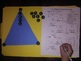# Multiplication Triads - Work Pages and Game - Multiplication Fact FamiliesSubject
Resource Type
File Type

PDF

(1 MB|35 pages)
Standards
• Product Description
• StandardsNEW
This 35 page booklet is the perfect tool to teach your students multiplication fact families from x1 through x12. The booklet includes a printable work mat, printable 12 sided dice (and cards so you can choose how students play the game), and teacher notes. The coordinating work pages can be used independently of the game for extra practice or homework. The game can be played with individual students, small groups of students or an entire class.

Need it hardcopy? You can now find this booklet on Amazon Prime!
You can search using key words "Multiplication Triads"

Or

Apply properties of operations as strategies to multiply and divide. Examples: If 6 × 4 = 24 is known, then 4 × 6 = 24 is also known. (Commutative property of multiplication.) 3 × 5 × 2 can be found by 3 × 5 = 15, then 15 × 2 = 30, or by 5 × 2 = 10, then 3 × 10 = 30. (Associative property of multiplication.) Knowing that 8 × 5 = 40 and 8 × 2 = 16, one can find 8 × 7 as 8 × (5 + 2) = (8 × 5) + (8 × 2) = 40 + 16 = 56. (Distributive property.)
Determine the unknown whole number in a multiplication or division equation relating three whole numbers. For example, determine the unknown number that makes the equation true in each of the equations 8 × ? = 48, 5 = __ ÷ 3, 6 × 6 = ?.
Interpret products of whole numbers, e.g., interpret 5 × 7 as the total number of objects in 5 groups of 7 objects each. For example, describe a context in which a total number of objects can be expressed as 5 × 7.
Total Pages
35 pages
N/A
Teaching Duration
N/A
Report this Resource to TpT
Reported resources will be reviewed by our team. Report this resource to let us know if this resource violates TpT’s content guidelines.